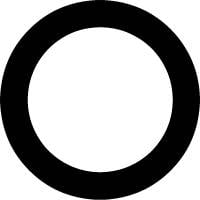# Thermal Expansion of a metal ring

## Homework Statement

A ring of aluminum has a hole in the middle. When the ring is heated:

a) the hole decreases in diameter
b) the aluminum expands outward and the hole remains the same size.
c) the area of the hole expands by the same percent as the area of the aluminum.
d) the area of the hole expands by a greater percent than the area of the aluminum.
e) the linear expansion causes the hole to expand in a slightly elliptical pattern.

## Homework Equations

ΔA=$\alpha$AΔT
$\alpha$=area thermal expansion coefficient
A=area
T=temperature

## The Attempt at a Solution

I know the hole gets larger. What I can't figure out is whether the hole expands by the same percent of the area of by a greater percent. I want to say that it will expand at a greater percent because the metal expands everywhere in one dimension but area is in two dimensions (if that makes any sense).
Like the area of the circle is ∏r2, but the area of the ring is ∏(R2-r2) with big R being the outer radius and little r being the inner radius. Because of this formula, wouldn't the inside expand by a greater amount than the outside.
I've been thinking about this problem so long I've kinda gotten burnt out at looking at it from any other angles...## The Attempt at a Solution

Chestermiller
Mentor
If 2πR and 2πr are the outer circumference and the inner circumference of the ring before the ring is heated, what are the inner and outer circumferences after it is heated? What are the inner and outer radii after it is heated? What is the area of the hole after the ring is heated? What is the area of the ring after it is heated?

Are those real questions or hints to get me going in the right direction?
I gave all the information given, this is a conceptual question...

I don't know how to compare the final to the initial, that's why I am having a hard time finding whether the answer is C) or D). I think I could be approaching the problem from the entirely wrong direction. Also there is very little information on these types of problems in my textbook.

(By no means do I intend to offend, I am pretty tired...)

haruspex
Homework Helper
Gold Member
2020 Award
c) the area of the hole expands by the same percent as the area of the aluminum.
d) the area of the hole expands by a greater percent than the area of the aluminum.
I want to say that it will expand at a greater percent because the metal expands everywhere in one dimension but area is in two dimensions (if that makes any sense).
It does, but the options c and d don't compare area of hole with linear expansion of ring; they compare it with area expansion of ring.

NascentOxygen
Staff Emeritus
Is it valid to imagine this drawing as being a painting on a square sheet of metal? Then address the question, how will this painted pattern change as the metal sheet warms?

Chestermiller
Mentor
Are those real questions or hints to get me going in the right direction?
I gave all the information given, this is a conceptual question...

I don't know how to compare the final to the initial, that's why I am having a hard time finding whether the answer is C) or D). I think I could be approaching the problem from the entirely wrong direction. Also there is very little information on these types of problems in my textbook.

(By no means do I intend to offend, I am pretty tired...)

They are real questions and hints to get you going in the right direction.

Let me get you started. If 2πR is the outer circumference before the ring is heated, then after the ring is heated, the outer circumference is $2\pi R (1+\beta \Delta T)$, where β is the linear coefficient of thermal expansion. The answers to the rest of my equations should be easy now.

Chet